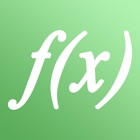High School Math - Calculus

• SEARCH TYPE

All iPhone applications categories

All iPhone games categories# High School Math - Calculus

10 Users
rating

## Screenshots

Description

The perfect high school & college app for math (calculus, solving equations and matrices) which combines theory and practice!

6 reasons to buy this app:
1. Choose from 20 topics
2. Easy step by step explanations
3. Examples with numbers/parameters
4. Infoboxes include more details
5. Over 40 math problems to train
6. Exact handwritten solutions

More detailed description:
• Detailed and colored Step by Step explanations and examples ensure a solid basic​ knowledge.
• Through switching between examples of numbers and parameters, you' ll master both types of math problems and lose the fear of evil letters in equations...
• Detailed information can be read in infoboxes.
• After you understood the theory, you are able to solve math problems, which are available directly in the app.
• Handwritten solutions are available to control yourself. It also teaches you how to solve and write down the math problems what avoids you giving away points in the exams.
• This is the only app which shows you how to find the equations of tangent lines, inflection points and much more. Nearly all other math apps are only able to show you how to solve an equation.

Topics:

DIFFERENTIAL CALCULUS
• Zeros of a Function
• Y-Intercept
• Intersection of two Functions
• Perpendicular
• Tangent with given Point
• Tangent parallel to given Line
• Tangent through Point not on Curve
• Turning Points
• Inflection Points
• Find the Equation of a Curve
• Locus Curve of a Family of Curves

SOLVE EQUATIONS
• Substitution
• Zero Product Property

INTEGRAL CALCULUS
• Integral Rules
• Calculate Integrals

SYSTEM OF LINEAR EQUATIONS
• Rules
• Put into Row Echelon Form
• Solvability

MATH PROBLEMS
• Practice with Solutions

The app is not a calculator. Check out our app bundle (located above the screenshots) that includes a full graphing calculator.

Also take a look at our other apps, which are useful for college & high school.

## Users review

from 8 reviews

"Awesome"

10